# vs.eyeandcontacts.com

## Chapter 14 Practical Geometry Exercise 14.2

Question 1: Draw a line segment of length 7.3 cm using a ruler.

Given
line segment length = 7.3 cm

Rough figure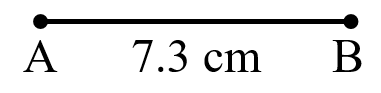Actual figureSteps of Construction
1. Mark Point A.
2. Place 0 cm at Point A.
3. At 7.3 cm, mark Point B.
4. Join the two point, A and B.
5. Therefore, AB is the line segment.

Question 2: Construct a line segment of length 5.6 cm using ruler and compasses.

Given
line segment length = 5.6 cm

Rough figure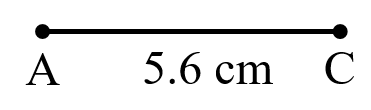Actual figure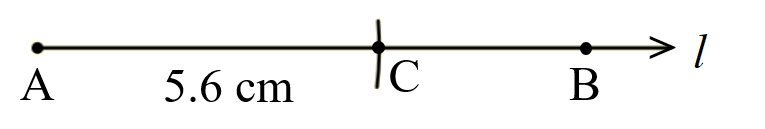Steps of Construction
1. Draw a line l. Mark point A on the line l.
2. Open the compass upto 5.6 cm.
3. With A as centre, radius 5.6 cm draw an arc to cut line l at C.
4. Therefore, AC is the required line segment.

Question 3: Construct line segment AB of length 7.8 cm. From this, cut off line segment AC of length 4.7 cm. Measure line segment BC.

Given
line segment AB length = 7.8 cm
line segment AC length = 4.7 cm

Rough figure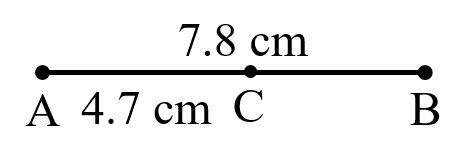Actual figure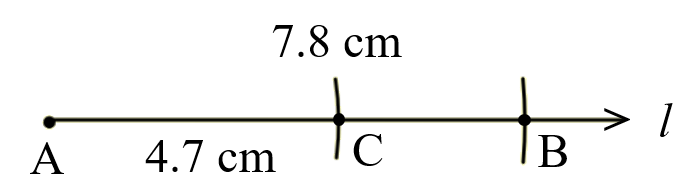Step of Construction
1. Draw a line l. Mark point A on the line l.
2. Open compass upto 7.8 cm.
3. With A as centre, radius 7.8 cm draw an arc to cut the line l at B.
4. Open the compass upto 4.7 cm
5. With A as centre, radius 4.7 cm draw an arc to cut the line segment AB at C.
6. Therefore, AB and AC are the required line segments.

When we measure the length of BC, we get 3.1 cm.

Question 4: Given line segment AB of length 3.9 cm, construct line segment PQ such that the length of line segment PQ is twice that of line segment AB. Verify by measurement. (Hint: Construct line segment PX such that length of line segment PX = length of line segment AB; then cut off line segment XQ such that line segment XQ also has the length of line segment AB.)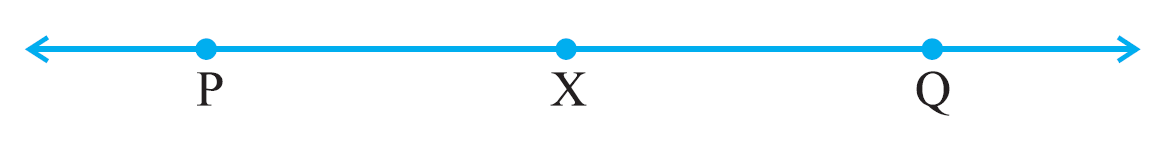Given
line segment AB length = 3.9 cm
line segment PQ is twice of line segment AB.

Rough figure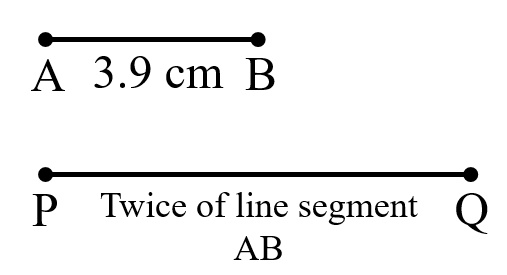Actual figure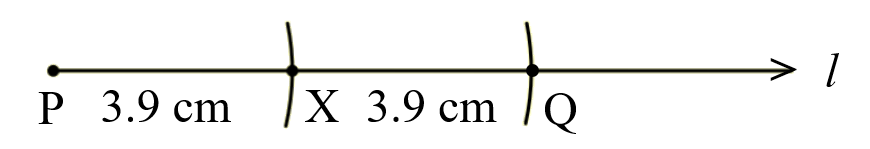Steps of Construction
1. Draw a line l. Mark point P on the line l.
2. Open compass upto 3.9 cm.
3. With P as centre, radius 3.9 cm draw an arc to cut the line l at X.
4. With X as centre, radius 3.9 cm draw an arc to cut the line l at Q.
5. Therefore, PQ is the required line segment.

Question 5: Given line segment AB of length 7.3 cm and line segment CD of length 3.4 cm, construct a line segment line segment XY such that the length of line segment XY is equal to the difference between the lengths of line segment AB and line segment CD. Verify by measurement.

Given
line segment AB length = 7.3 cm
line segment CD length = 3.4 cm

Rough figure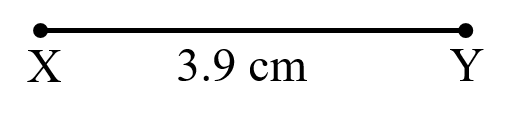Actual figure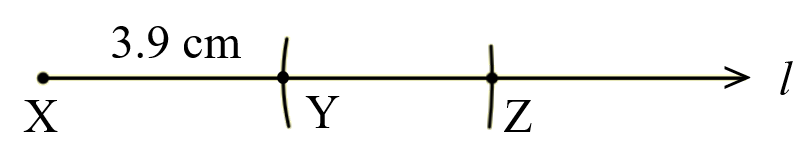Steps of Construction
1. Draw a line l. Mark point X on the line l.
2. Open compass upto 7.3 cm.
3. With X as centre, radius 7.3 cm draw an arc to cut line l at Z.
4. Open compass upto 3.4 cm.
5. With Z as centre, radius 3.4 cm draw an arc to cut line segment XZ at Y.
6. XY is the required line segment.

By measurement, the length of line segment XY = 7.3 cm - 3.4 cm = 3.9 cm